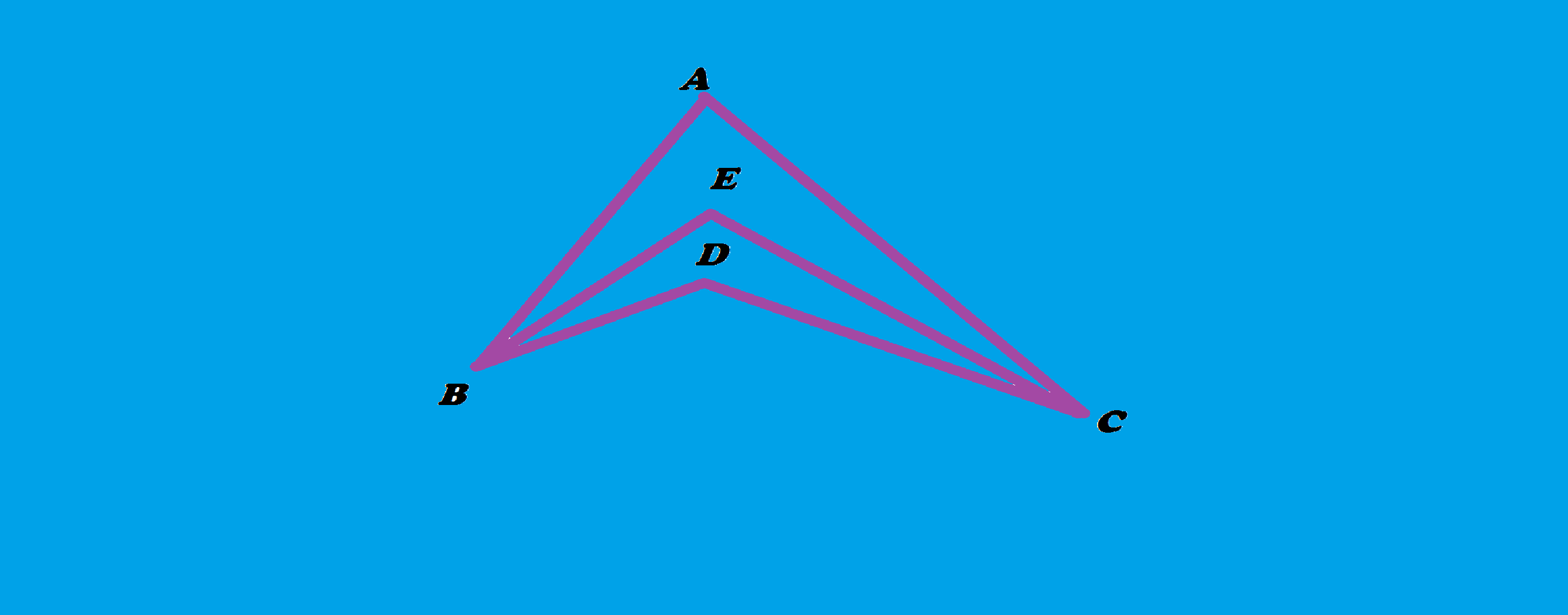Angles.

Geometry Level 1In the figure above, $\angle ABE$ = $\angle ECD$ and $\angle EBD$ = $\angle ACE$ . If $\angle BAC = 80 ^ \circ$ and $\angle BEC = 100 ^ \circ$ then, what is the value of obtuse $\angle BDC$.

×